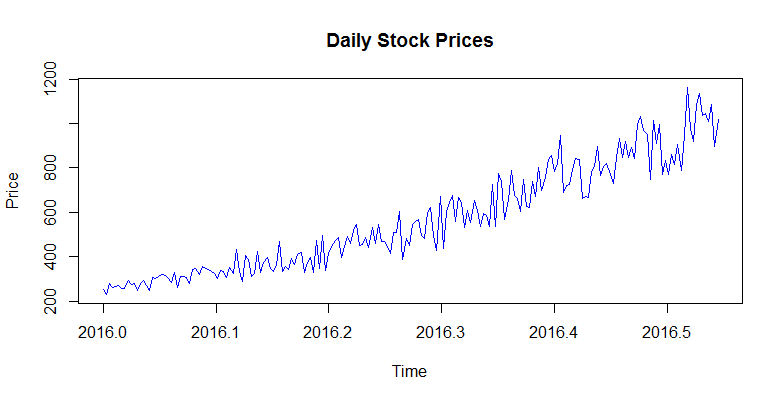# Time Series Transformation in R

We will now learn about how we can perform the mathematical transformations in R in order to make a non-stationary series stationary.

We have the daily stock prices of an imaginary stock exhibiting rapid growth stored in a csv file. You can download the csv file and follow along this tutorial to perform the transformations in your R console. There are two hundred observations starting from 1st Jan , 2016.

We have placed the csv file in our working directory as shown below:

> setwd("C:/Users/Manish/Dropbox/Finance Train/Courses/Data")
> getwd()
 "C:/Users/Manish/Dropbox/Finance Train/Courses/Data"
> list.files()
 "stock_daily.csv"


As you can see, the file stock_daily.csv in available in our working directory.

We will read this csv file in R using read.csv() function and store the dataset in a variable called sp. The data will be stored as a dataframe.

> sp <- read.csv("stock_daily.csv", header=FALSE)
>
> str(sp)
'data.frame':    200 obs. of  1 variable:
\$ V1: num  254 229 277 262 264 ...
>


### Convert dataframe to time series using ts() function

Now that we have the data in a dataframe, we can convert it into a time series object using the ts() function as shown below:

> sp_ts <- ts(sp, start=2016, frequency=365)


### Plot the series

Let's now plot the time series using the plot.ts() function.

> plot.ts(sp_ts,col=4, main="Daily Stock Prices", ylab="Price")We can observe two things here:

1. There is a clearly visible upward trend in the data
2. The variability in the data is increasing over time.

Let's now look at how we can remove both these patterns to stationarize the series.